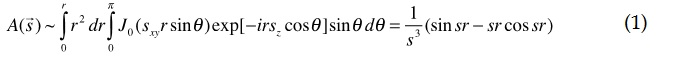# Abstract P-50: On the Features of X-Ray Laser Diffraction on Certain Elements of Macromolecular Structures

Konstantin V. Shaitan

International Journal of Biomedicine.2019;9  Suppl_1:S39-S39.
http://dx.doi.org/10.21103/IJBM.9.Suppl_1.P50
Originally published June 29, 2019

Abstract:

Background: The possibility of using free electron X-ray lasers for solving problems of structural biology in single particle experimental mode is discussed. In the first Born approximation analytical expressions for the amplitudes and intensities of X-ray diffraction on cylinders, balls, hemispheres and tori in various combinations and orientations are obtained. The characteristic diffraction patterns from these geometric bodies are analyzed. The modeling of elements of the secondary structure of biopolymers (for example, alpha-helices, characteristic DNA packs, some viruses, etc.) is discussed by a certain set of these geometric bodies with the corresponding parameters.
Methods: As an example (due to space constraints and because of the reason below), we present the solution of the scattering equation in the Born approximation for the amplitude of diffraction on a hemisphere with a uniformly distributed density. Note, that the solution of a similar problem for a sphere (ball) is well knownbut it is difficult to find the analytical results for hemispheres and many other geometric bodies. The solution for a sphere (ball) can be obtained from the next integralThe calculation of the integral of type (1) for a hemisphere turns out to be much more complicated. Available reference books give this integral or integrals to which it can be reduced with errors.
Results: We present the correct value of the integral, which is expressed in terms of Bessel function and Lommel function U0 (x, y) of two argumentsHere we have introduced the standard notation for the modulus of the scattering vector and introduced the notation sz for the projection of the scattering vectoron the axis of symmetry of the hemisphere. Considering the corresponding integrals, one can also obtain a useful relation for Lommel functionConclusion: Using (4) it is easy to obtain a formula for the scattering amplitude of a ball made up of two halves with different densities. Fig.1.1 demonstrates the pattern which is typical for uniform ball at any orientation angle as well. But in the case of the hemisphere (and heterogeneous balls) the diffraction patterns form some characteristic features depend on the orientation angles.